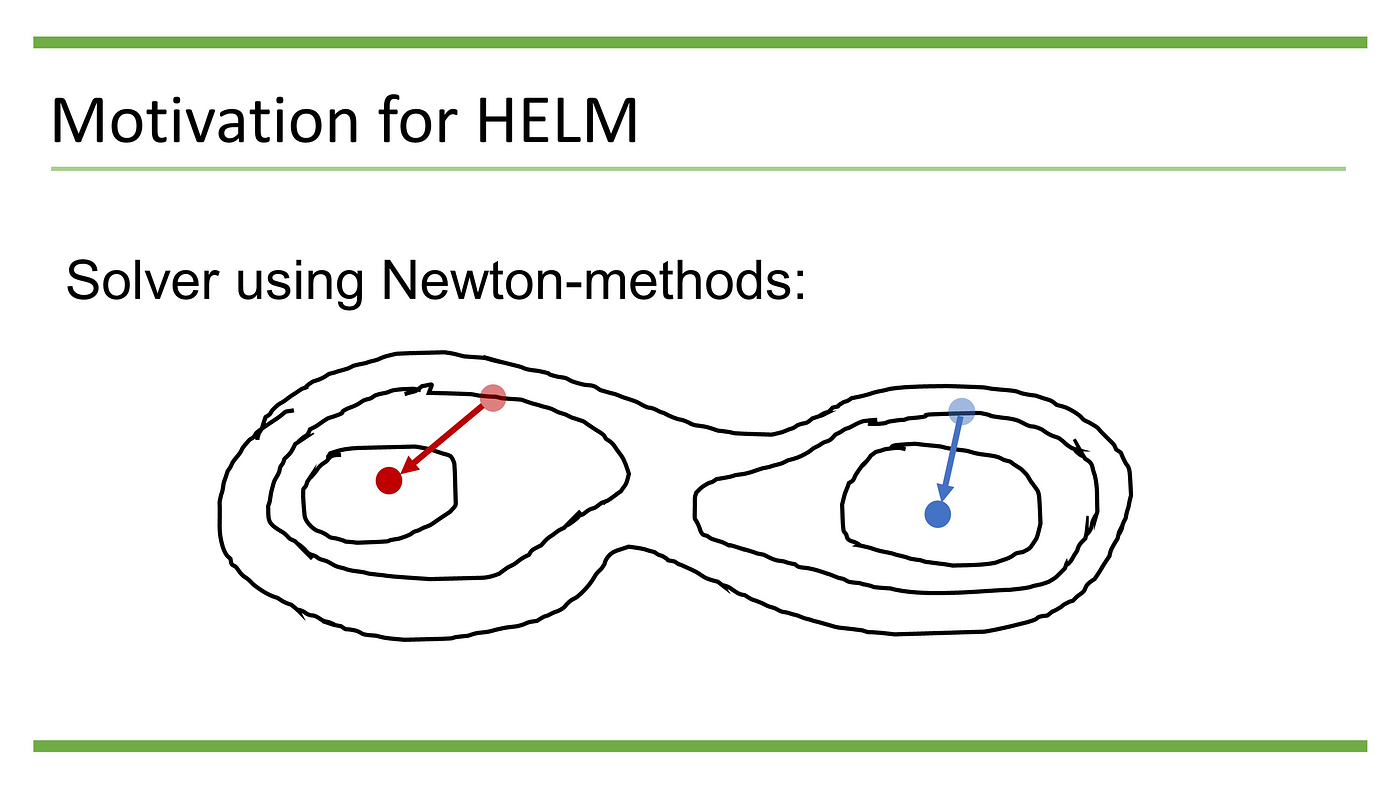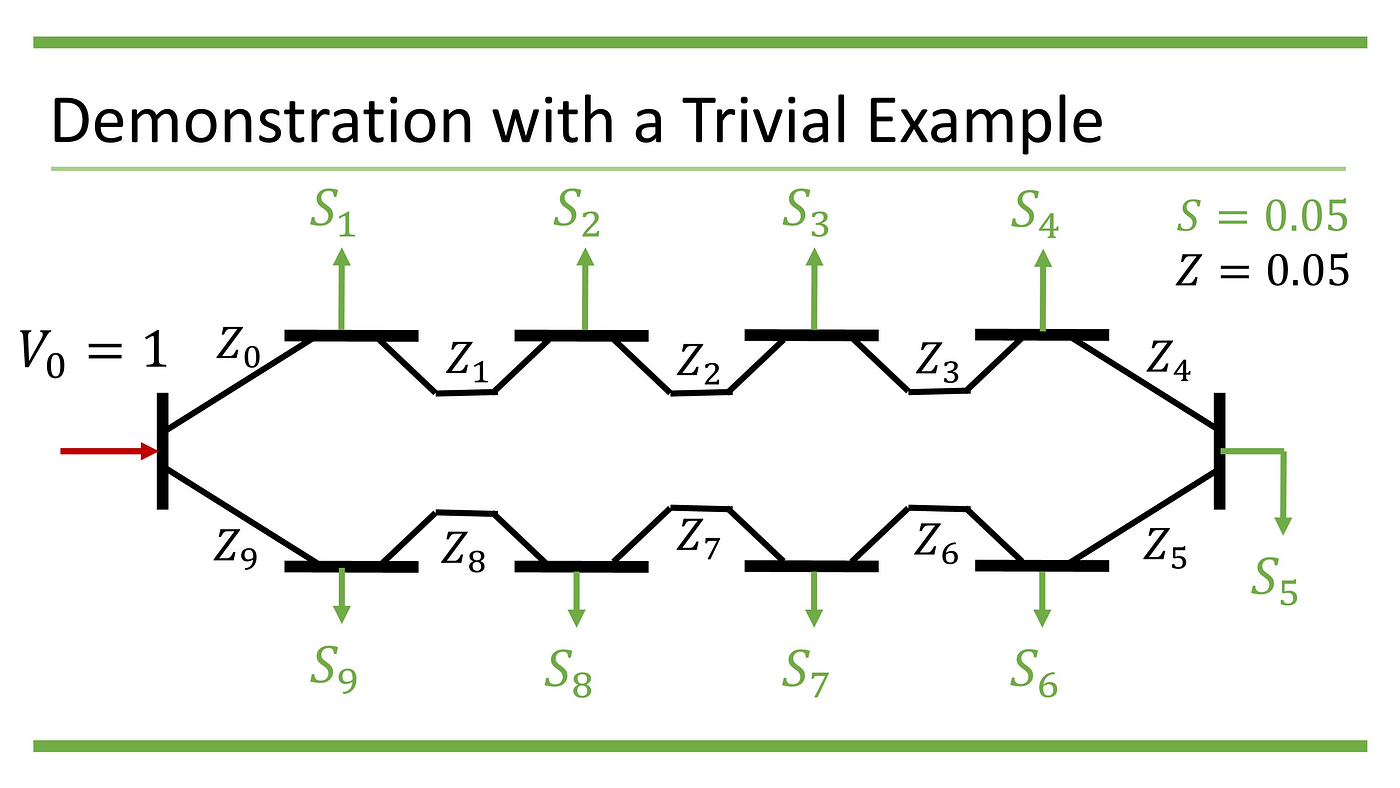# Motivation for HELM

As we have shown in the first part of the series, one of the feature and also the main problem in power flow analysis is that the power flow equation

is not linear.

Nonlinear equations can be solved using Newton method. However, Newton method is sensitive to the initial guess of the solution; it therefore may not always yield the desired high voltage solution.Newton method is sensitive to the initial guess of the solution

# Properties of the Power Flow Equation

To find a method that can univocally yield the high voltage solution whenever such solution exists, let us look deeper into the properties of the power flow equation.

## 1. Node admittance matrix [Y_eq] has rank n -1

For a well-connected power network, the node admittance matrix [Y_eq] has rank n -1, where n is the number of nodes in the power system.

This means that there is a non-zero vector {η} such that

So that the power flow equation will always have a non-zero trivial high voltage solution.

To solve the power flow equation, given [Y_eq] only has rank n-1, we usually choose a node whose voltage is a pre-determined reference value, thereby reducing the number of unknown variables from n to n — 1. This node is usually called the “slack bus”.

## 2. Power flow equation is scalable

If {V} is the solution to the equation

Then {U}=α{V} is the solution to the equation

Therefore, by rescaling the node admittance matrix [Y_eq], the voltage value of the slack bus is arbitrary. It is usually set to 1 (per unit).

# Main Idea of HELM

If we parameterized the power flow equation as

Then lambda = 0 corresponds to a trivial high voltage solution (for example, when all the nodes have the same voltage as the slack bus), whereas lambda = 1 corresponds to the high voltage solution we actually want.

The main idea of HELM is that if we can embed the power flow equation into algebraic equations of holomorphic (analytical complex) functions, then as lambda goes from 0 to 1, the series approximation of the voltage will converge univocally to the desired high voltage solution, whenever such solution exists.

This univocality comes from the fact that within the radius of convergence, holomorphic functions can be approximate arbitrarily close to the true value with series representation.

If all the holomorphic functions embedding the voltage has a radius of convergence greater than 1 at lambda = 0, the series converges at lambda = 1; otherwise it diverges.

# Workflow of HELM

## 1. Holomorphic embedding of the voltage

So the following set of equations are holomorphic:

## 2. Power series representation of the voltage

The second step is the power series representation of the voltage. The form of the power series depends on the type of nodes in the power network. In the simplest case, all nodes other than the slack bus are PQ-buses — i.e., real and reactive power at the nodes are given.

## 3. Term by term matching

The third step of HELM is term by term matching of the power series.

Notice that we already know {v_0} and {v hat_0} when k = 1, so {v_1} and {v hat_1} can be solved directly from the 2 equations.

Again {v_0}, {v_1}, {v hat_0}, and {v hat_1} are all known when k = 2, so {v_2} and {v hat_2} can be solved directly from these 2 equations. This process can continue for arbitrarily large k.

## 4. Near-diagonal Padé approximant

When the power system is near the bifurcation point, the power series can converge / diverge slowly. Construction of a near-diagonal Padé approximant from the original power series can give us a more robust indication whether the series converges or not.

If there exists a root in the denominator of the near-diagonal Padé approximant whose magnitude is smaller or equal to 1, then the original power series diverges.

# Demonstration with a Trivial Example

Let us demonstrate the concepts presented before by a trivial example. This is a ring structure micro-grid of 10 nodes, with the zeroth node being the slack bus. Each node draws the same power S = -0.05 (pu) from the grid and each line has the same impedance. We run the HELM with 20 terms of the power series.The micro-grid in the trivial example

If you run the c++ code here, you will get the following results.

In the first part of the output, we can see the resulting voltage by directly summing up all the coefficients of the power series. It is difficult to tell whether or not the series diverges with this result.

In the second part of the output, we can see that the Padé approximant of the voltage yields slightly lower values compared with direct summation. The Padé approximant converges to the high voltage solution faster than direct summation whenever such solution exists, but it may still converge even when a high voltage solution does not exist.

In the third part of the output, we can see that there is a root in the denominator of the Padé approximant whose magnitude is smaller than 1, this implies that the power series diverges and there is no solution to the original power flow equation.

Indeed; if we increase the number of terms of the power series to 100, we can start seeing the result of the direct summation diverges. Meanwhile, with 100 terms the Padé approximant of the voltage still does not diverge, but there will be more poles whose magnitude is smaller than 1.

# Potential Problems When Using HELM

## 1. Computational burden of finding the roots

Calculating the Padé approximant only makes sense when the roots in the denominator of the approximant are also calculated. Root searching of high order polynomials incurs additional computational burden.

## 2. Spurious poles of Padé approximant

If the number of power terms is very large, numerical errors of calculating the Padé approximant may lead to spurious poles. Sophisticated methods exist to “clean up” the spurious poles.

--

--## Tony Yen

A Taiwanese student who studied Renewable Energy in Freiburg. Now studying smart distribution grids / energy systems in Trondheim.